Go Back

# How to Compare 2 Columns in Excel

Data reconciliation is a very important task that we do while analyzing data in Excel. Comparing 2 columns or comparing 2 sets of data in Excel is a very common practice and there are a number of different ways to do this, depending upon expertise and the nature of requirements.
Generally, we compare columns in Excel to find matches and differences. However, it’s sometimes difficult to choose the best method due to data layout and requirements. In this article, we will discuss different methods or techniques to compare 2 columns in Excel with examples and explanation, along with best practices.

### Compare 2 columns using VLOOKUP

The VLOOKUP function is used to compare two columns in Excel based on exact and partial match type. This function is designed to compare columns, and to lookup the data vertically as its name is derived from Vertical Lookup. Make sure the values in both columns are unique. Otherwise VLOOKUP will pick an only first match and will ignore the second occurrence of the lookup value.

The syntax of VLOOKUP function is as follows;

`=VLOOKUP (lookup_value, table_array, column_index_number, [range-lookup])`

By column_index_number we can retrieve the information related to lookup_value in the 2nd column where match found.

Suppose we have 2 columns of Invoice Numbers where 1st column needs to compare with 2nd column, and VLOOKUP function returns those invoice numbers that are found in the 2nd column, on the basis of column-index_number and exact match type (Range_lookup). We will use this formula to do that.

`=VLOOKUP(A2,\$B\$2:\$B\$17,1,FALSE)`

Here, we will match each invoice number of the 1st list in column A with the 2nd list in column B and VLOOKUP will return the lookup_value of the 1st list that is found in the 2nd list. If lookup_value is not found in the 2nd list, VLOOKUP function will return a #NA error.

Please have a look at how this comparison is performed using the VLOOKUP function as per above formula.
Here,

A2 is cell reference of lookup_value of 1st list

\$B\$2:\$B\$17 is table_array of 2nd list

1 is column_index_number as there is one column in table_array from which the matching value must be returned.

FALSE is range_lookup for exact match type

We will insert the formula in an active cell, like in C2 and will copy down to other cells corresponding to lookup-values of the 1st list in column A, as shown below.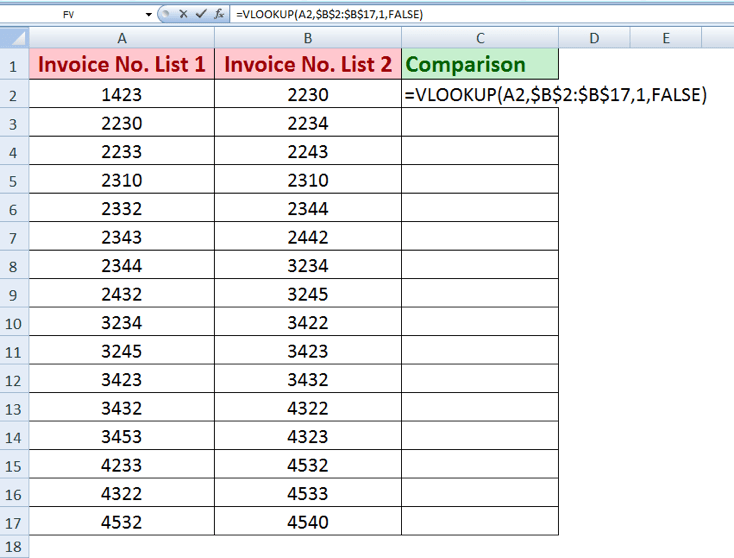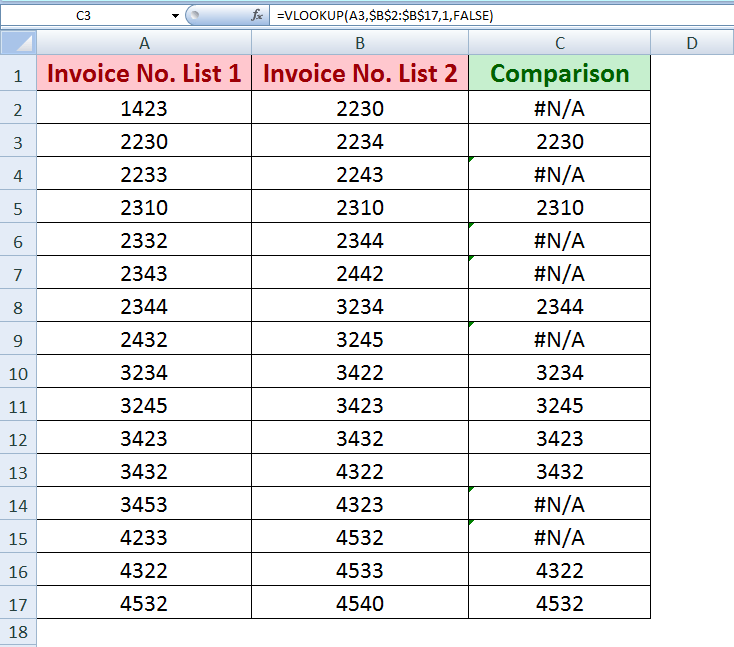Hence, VLOOKUP function will return all those invoice numbers of list 1 that are present in List 2, and will return #NA error that is not found in List 2.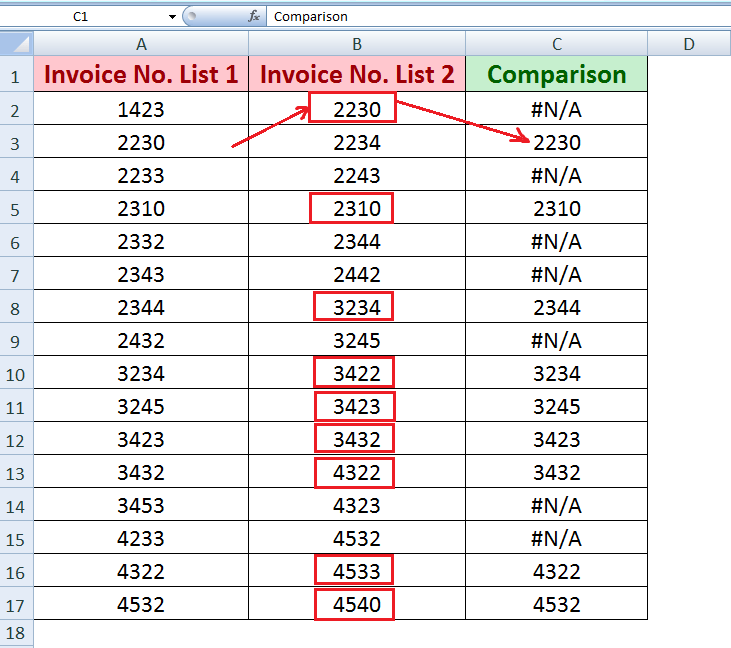### Compare 2 columns using MATCH

The Excel MATCH function searches for a value in a range of cells (array) and returns the relative position of that value in that range. We can easily compare 2 columns in Excel using the MATCH function based on exact and partial match types.

The syntax for MATCH function in Excel is;

`MATCH ( value, array, [match_type] )`

As we have two lists of invoices in our examples here, so the value is the lookup value from the 1st list and array is the range of cells in the 2nd list, and match_type is exact.

The formula to compare 2 columns using MATCH function is as follows.

`=MATCH(A2,\$B\$2:\$B\$17,0)`

This formula will return the relative position of matching the value in an array instead of the value itself and will return #NA error where there is no match. Apply this formula in the first active cell and then copy it down to other corresponding cells of the list.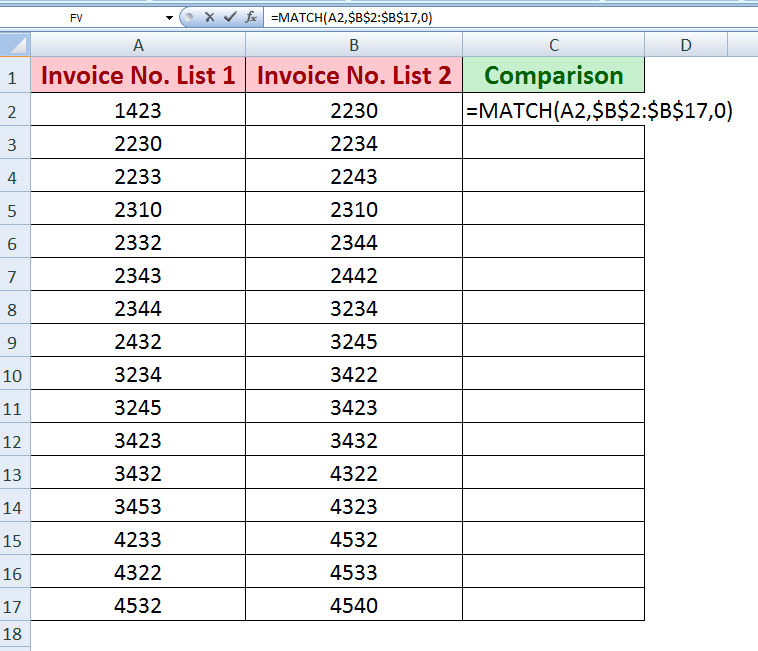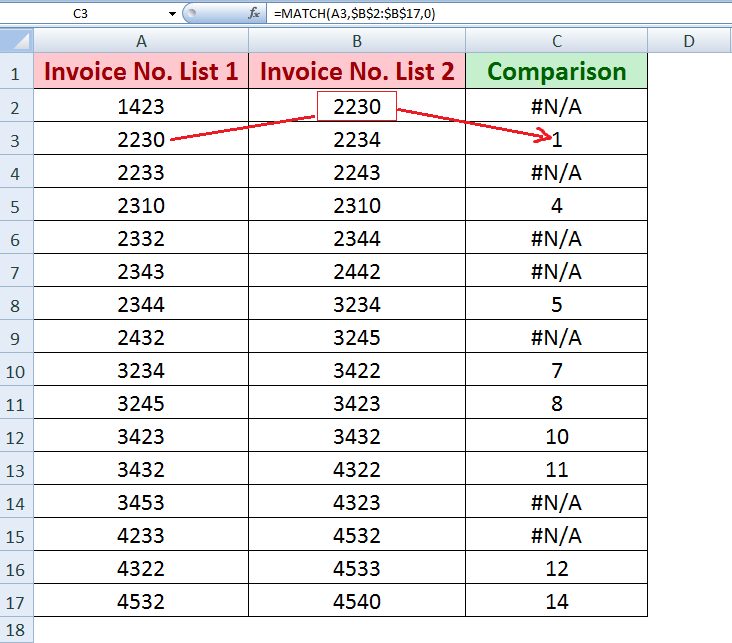Here, you can see MATCH function has returned #NA error for 1st invoice number and returned relative position of 2nd invoice number in list2.

### Compare 2 columns using COUNTIF

COUNTIF function in Excel is also used to compare 2 columns data by counting the occurrence of a value in a range of cells.

In this example, we can identify whether an invoice number is present in the 2nd column or not. If it returns 0 then there is no match, else the value has a match.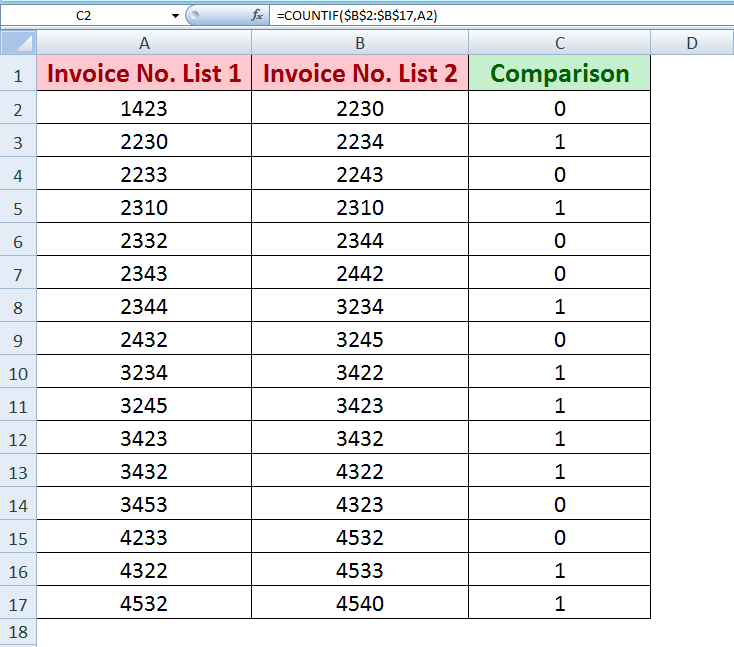Combining IF and COUNTIF functions we can return the specified result if the given logical test is TRUE or FALSE, such as

If COUNTIF(\$B\$2:\$B\$17,A16)>0, then return “Matched“, else return FALSE

Here is the formula to do that.

`=IF(COUNTIF(\$B\$2:\$B\$17,A2)>0,"Matched")`

This will return the specified result “Matched” if the given logical test returns TRUE, else it will return FALSE.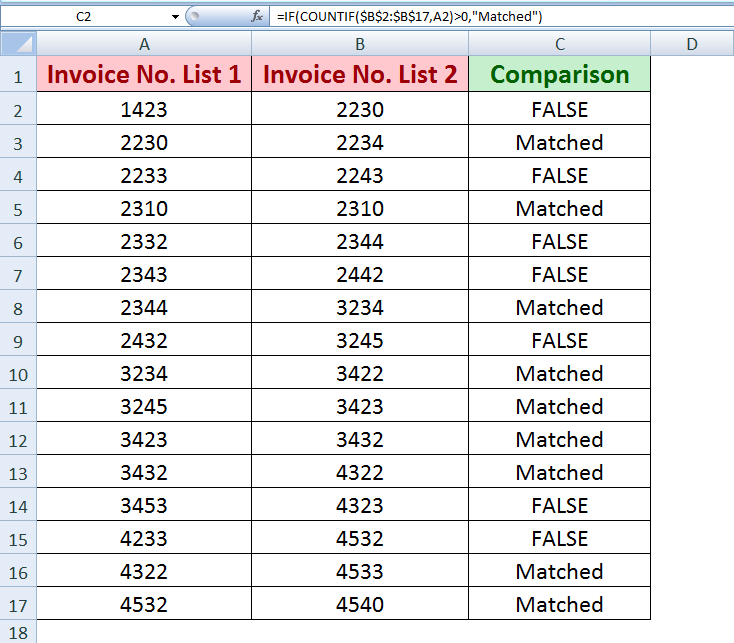### Compare 2 columns using INDEX, MATCH

Using a combination of INDEX, MATCH functions in Excel we can compare 2 columns data and also retrieve the matching values as VLOOKUP function does.

As we know that MATCH function returns the relative position of matched value, so we can use that relative position for row and column position required in INDEX function to retrieve the matching values in both columns, such as;

`=INDEX(\$B\$2:\$B\$17,MATCH(A2,\$B\$2:\$B\$17,0))`

As the data in both columns are laid out in rows, so we will use MATCH function relative position for row number and will ignore for column number. This formula returns all those values from the 2nd column where MATCH function returns the relative position of 1st column values in the 2nd column as shown below.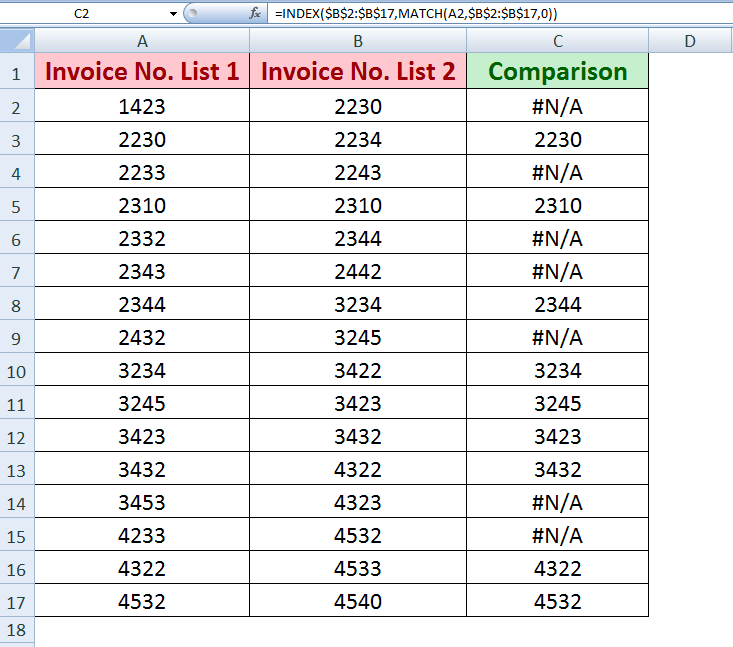### Compare 2 columns using Conditional Formatting

Conditional Formatting is another option in Excel to compare 2 columns of data. We can highlight unique or duplicate values found in 2 columns. Here is the simplest way to do that;

Press CTRL key on the keyboard and select data of both columns. Click on Conditional Formatting feature from the Styles section at Home tab. From Highlight Cells Rules, choose Duplicate Values. A duplicate values dialog box appears, and you can format cells that contain duplicate or unique values from a drop-down list with available formatting styles list, or you can choose to customize style to highlight those cells. Press OK to confirm that changes.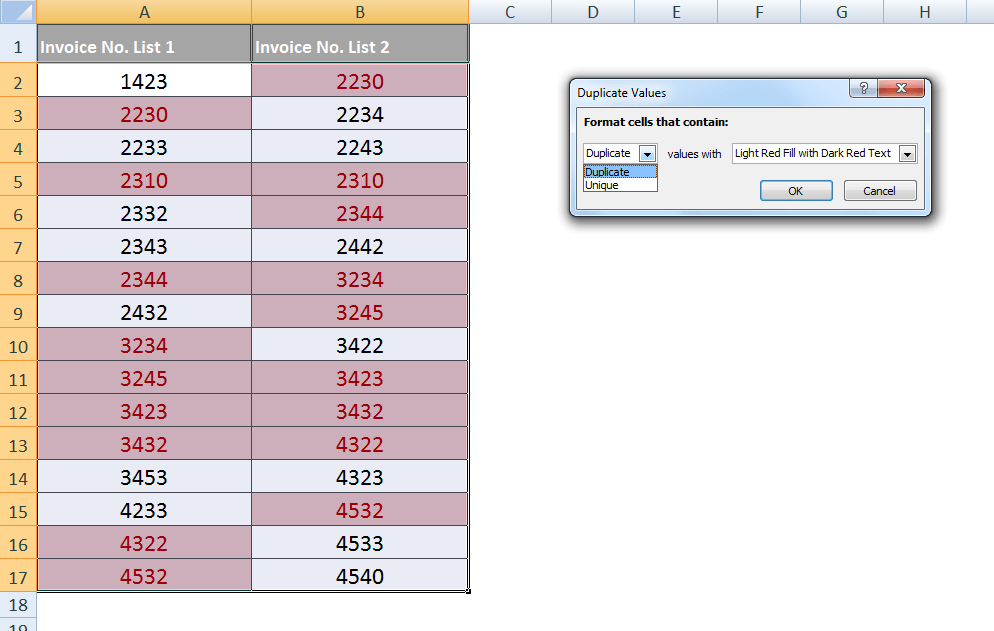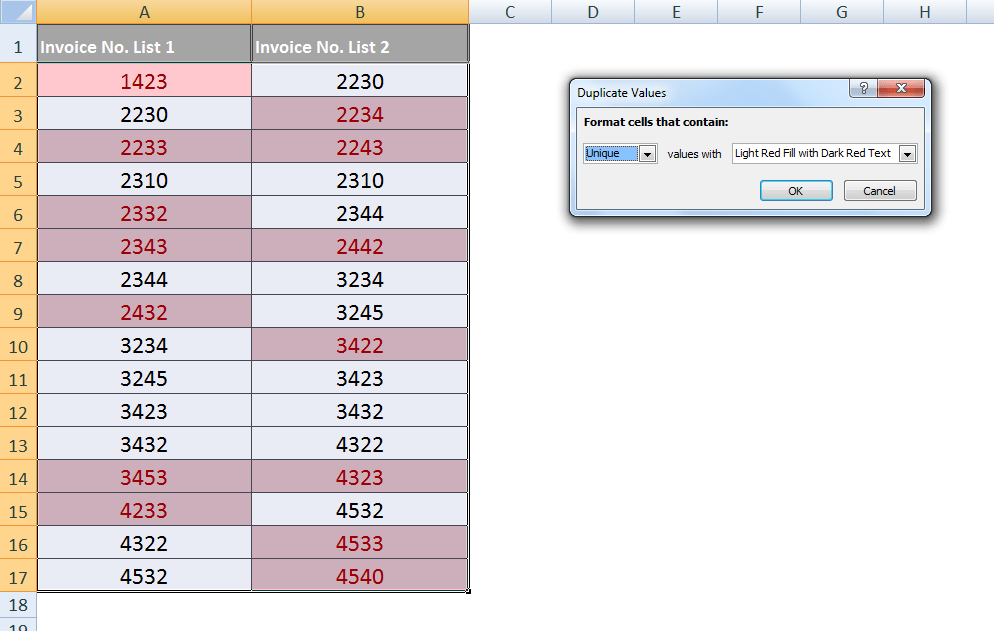The problem you are trying to solve could be more complex, if you are in a rush, or need a real Expert to help you save hours of struggle, click on this link to enter your problem and get connect to a qualified Excel expert in a few seconds. You can share your file, and an expert will create a solution for you on the spot during a 1:1 live chat session. The first session is free.

### Did this post not answer your question? Get a solution from connecting with the expert.

Another blog reader asked this question today on Excelchat:
Here are some problems that our users have asked and received explanations oncompare with graphs
Solved by X. S. in 16 minscompare chart
Solved by G. W. in 20 minsCan you compare call rates and recalulate these based on time then compare the the values to calculate a credit?
Solved by V. S. in 27 mins